In :
``````#Setting working directory
import os
os.chdir('E:\\Imarticus\\DSP17\\final')
print (os.getcwd())``````
```E:\Imarticus\DSP17\final ```
In :
``````# Import necessary libraries
import matplotlib.pyplot as plt
import pandas as pd
import numpy as np
import seaborn as sns
%matplotlib inline
from IPython.display import Image``````
In :
``````#------------#
# Some Basics
#------------#

# kNN and Decision Trees are believed to be two most simple and intuitive Algos
# They are simple to understand and easy to explain

# KNN can be used for both classification and regression predictive problems.
# Find k Nearest Neighbors and take the majority vote or average as applicable
# If you would like to substitute a missing value, (whether continuous or
# Categorical) kNN might work best in some cases
# It is also very useful in building recommender systems

# kNN is also called a Lazy Learner
# A lazy learner, on the other hand, does not build any model beforehand; it waits for
# the unclassified data and then winds it way through the algorithm to make classification
# prediction. Lazy learners are, therefore, time consuming–each time a prediction is to be
# made all the model building effort has to be performed again. We will see!

# KNN algorithm fairs across all parameters of considerations.
# It is commonly used for its easy of interpretation and low calculation time.
# See below:
Image('KNN comparison.png')``````
Out: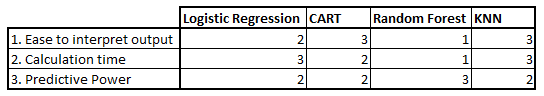In [ ]:
`` ``
In [ ]:
`` ``
In :
``````#==============================#
# Generic steps to KNN Modeling
#==============================#

# Load the data and clean/transform/standardize as applicable
# Initialise the value of k
# For getting the predicted class, iterate from 1 to total number of training data points
# Calculate the distance between test data and each row of training data.
# Here we will use Euclidean distance as our distance metric since it’s
# the most popular method and default one in many implementations.
# for 'n' data points, it is almost n^2 computations you do
# Add the distance and the index of the example to an ordered collection
# Sort the calculated distances in ascending order based on distance values
# Get top k rows from the sorted array (k is the number of nearest neighbors needed)
# Get the labels of those selected k entries
# If regression, return the mean of the K labels
# If classification, return the mode of the K labels

# k-NN does not require an explicit training step. In k-nearest neighbor algorithm,
# the example data is first plotted on an n-dimensional space where ‘n’ is the number
# of data-attributes. Each point in ‘n’-dimensional space is labeled with its class value.

# To discover classification of an unclassified data, the point is plotted on this n-dimensional
# space and class labels of nearest k data points are noted. Generally k is an odd number.

# In the generic k-NN model, each time a prediction is to be made for a data point,
# first this data point’s distance from all other points is to be calculated and then
# only nearest k-points can be discovered for voting. This approach is also
# known as brute-force approach.

# Python's SKLEARN implementation is a bit optimised:
#---------------------------------------------------

# When the volume of data is huge and its dimension is also very large, say, in hundreds
# or thousands, this repeated distance calculations can be very tedious and time consuming.
# To fasten up this process and so as to avoid measuring distances from all the points in
# the data set, some prepossessing of training data is done. This per-processing helps to
# search points which are likely to be in its neighborhood.
# So, It may not be necessary to compute all n^2 pairs of distances.
# Instead, you can use a Kd tree or a ball tree (both are data structures for
# efficiently querying relations between a set of points).

# Scipy has a package called scipy.spatial.kdtree.
# It does not currently support hamming distance as a metric between points.
# However, sklearn do have an implementation of ball tree with hamming distance supported.
# Here's a small example using sklearn's ball tree.
# link: https://stackoverflow.com/questions/40733497/optimize-hamming-distance-python``````

## kNN for Classification

In :
``````# Read the data
df = pd.read_csv('knn_Classified Data.txt', index_col=0)
df.head()
``````
Out:
In :
``df.shape``
Out:
``(1000, 11)``
In :
``````# Import module to standardize the scale
from sklearn.preprocessing import StandardScaler
# Create instance (i.e. object) of the standard scaler
scaler = StandardScaler()
# Fit the object to all the data except the Target Class
# use the .drop() method to gather all features except Target Class
# axis -> argument refers to columns; a 0 would represent rows
scaler.fit(df.drop('TARGET CLASS', axis=1))``````
Out:
``StandardScaler(copy=True, with_mean=True, with_std=True)``
In :
``````# Use scaler object to conduct a transforms
scaled_features = scaler.transform(df.drop('TARGET CLASS',axis=1))
# Review the array of values generated from the scaled features process
scaled_features
# Notice that we have normalized our dataset minus the target column``````
Out:
``````array([[-0.12354188,  0.18590747, -0.91343069, ..., -1.48236813,
-0.9497194 , -0.64331425],
[-1.08483602, -0.43034845, -1.02531333, ..., -0.20224031,
-1.82805088,  0.63675862],
[-0.78870217,  0.33931821,  0.30151137, ...,  0.28570652,
-0.68249379, -0.37784986],
...,
[ 0.64177714, -0.51308341, -0.17920486, ..., -2.36249443,
-0.81426092,  0.11159651],
[ 0.46707241, -0.98278576, -1.46519359, ..., -0.03677699,
0.40602453, -0.85567   ],
[-0.38765353, -0.59589427, -1.4313981 , ..., -0.56778932,
0.3369971 ,  0.01034996]])``````
In :
``````# Let us now create a dataframe of scaled features
df_scaled = pd.DataFrame(scaled_features, columns = df.columns[:-1])
df_scaled.head()
``````
Out:
In :
``````# Let us now split the scaled data into training set and test set
# Import module to split the data
from sklearn.model_selection import train_test_split
# Set the X and ys
X = df_scaled
y = df['TARGET CLASS']
X_train, X_test, y_train, y_test = train_test_split(X, y, test_size=0.25, random_state=555)``````
In :
``````# We are now ready to train the kNN model
# Import module for KNN
from sklearn.neighbors import KNeighborsClassifier
# Create KNN instance
knn = KNeighborsClassifier(n_neighbors=1)
# n_neighbors argument represents the k value, i.e. the amount of neighbors used to ID classification
# n_neighbors=1 means each sample is using itself as reference, that’s an overfitting case

# Fit (i.e. traing) the model
knn.fit(X_train, y_train)
``````
```--------------------------------------------------------------------------- NameError Traceback (most recent call last) <ipython-input-1-1ddeeae8493a> in <module> 8 9 # Fit (i.e. traing) the model ---> 10 knn.fit(X_train, y_train) NameError: name 'X_train' is not defined```
In :
``````# Notice metric='minkowski', which represents the distance metric
# The Minkowski distance is a metric in a normed vector space which can be considered
# as a generalization of both the Euclidean distance and the Manhattan distance.
# when p=1, minkowski represents manhattan distance (L1) and
# when p=2, minkowski represents Euclidian distance (L2)``````
In :
``````# Now that the model is built, let us use ir for prediction
pred = knn.predict(X_test)
# Review the predictions
pred
``````
Out:
``````array([0, 0, 0, 1, 0, 0, 0, 1, 0, 0, 1, 1, 1, 0, 1, 1, 1, 0, 0, 1, 0, 0,
1, 0, 1, 0, 0, 0, 0, 0, 1, 0, 1, 1, 1, 1, 1, 0, 0, 0, 1, 0, 1, 1,
1, 0, 0, 1, 1, 1, 1, 0, 0, 1, 1, 1, 1, 0, 1, 0, 0, 1, 1, 1, 1, 1,
0, 1, 1, 0, 1, 1, 1, 0, 1, 0, 1, 0, 1, 1, 0, 0, 0, 0, 0, 1, 1, 1,
1, 0, 0, 1, 1, 1, 0, 1, 1, 0, 1, 1, 1, 0, 0, 1, 0, 0, 0, 1, 0, 1,
1, 0, 0, 1, 1, 0, 1, 1, 1, 0, 0, 0, 1, 0, 0, 1, 0, 1, 1, 0, 1, 0,
1, 0, 0, 0, 0, 0, 1, 1, 0, 0, 0, 0, 1, 0, 1, 0, 0, 1, 1, 0, 0, 0,
1, 0, 1, 1, 1, 1, 0, 1, 1, 0, 1, 1, 0, 1, 1, 0, 0, 0, 1, 1, 0, 1,
1, 1, 0, 1, 1, 1, 0, 0, 0, 1, 1, 0, 0, 0, 0, 0, 0, 1, 0, 1, 1, 0,
0, 1, 1, 0, 1, 1, 1, 0, 1, 0, 0, 0, 1, 0, 1, 0, 1, 1, 1, 1, 1, 0,
1, 0, 0, 0, 1, 0, 0, 1, 1, 1, 0, 0, 0, 1, 0, 1, 0, 0, 1, 0, 0, 1,
1, 1, 0, 1, 0, 1, 1, 1], dtype=int64)``````
In :
``````# Its time to evaluate our model
# Import classification report and confusion matrix to evaluate predictions
from sklearn.metrics import classification_report, confusion_matrix
# Print out classification report
print(classification_report(y_test, pred))
``````
``` precision recall f1-score support 0 0.90 0.86 0.88 127 1 0.86 0.90 0.88 123 micro avg 0.88 0.88 0.88 250 macro avg 0.88 0.88 0.88 250 weighted avg 0.88 0.88 0.88 250 ```
In :
``# This tells us our model was 88% accurate``
In :
``````# Print out confusion matrix
cmat = confusion_matrix(y_test, pred)
#print(cmat)
print('TN - True Negative {}'.format(cmat[0,0]))
print('FP - False Positive {}'.format(cmat[0,1]))
print('FN - False Negative {}'.format(cmat[1,0]))
print('TP - True Positive {}'.format(cmat[1,1]))
print('Accuracy Rate: {}'.format(np.divide(np.sum([cmat[0,0],cmat[1,1]]),np.sum(cmat))))
print('Misclassification Rate: {}'.format(np.divide(np.sum([cmat[0,1],cmat[1,0]]),np.sum(cmat))))``````
```TP - True Negative 109 FP - False Positive 18 FN - False Negative 12 TP - True Positive 111 Accuracy Rate: 0.88 Misclassification Rate: 0.12 ```
In :
``````# For determing the optimum value of k, you shoud ideally do multiple iterations while
# training and then test it with testing data.
# To select the K that’s right for your data, we run the KNN algorithm several times with
# different values of K and choose the K that reduces the number of errors we encounter
# while maintaining the algorithm’s ability to accurately make predictions when it’s given
# data it hasn’t seen before.

# Let us now evaluate the alternative k Values

# Let us create an empty list to capture the errors
error_rate = []
# Let us try the k values from 1 to 24
for i in range(1,25):
knn = KNeighborsClassifier(n_neighbors=i)
knn.fit(X_train, y_train)
pred_i = knn.predict(X_test)
error_rate.append(np.mean(pred_i != y_test))
``````
In :
``````# It will be good to plot error rate over different k values
plt.figure(figsize=(10,5))
plt.plot(range(1,25), error_rate, color='blue', linestyle='dashed', marker='o', markerfacecolor='red', markersize=10)
plt.title('Error Rate vs. K-Values')
plt.xlabel('K-Values')
plt.ylabel('Error Rate')``````
Out:
``Text(0, 0.5, 'Error Rate')``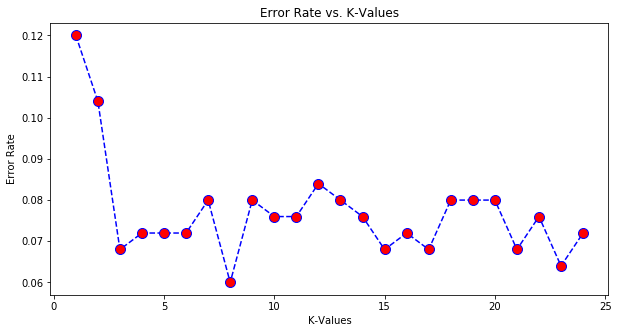In :
``````# We will retrain the model with an optimum k-value that we got from the above graph
knn = KNeighborsClassifier(n_neighbors=8)
knn.fit(X_train, y_train)
pred_new = knn.predict(X_test)
# Print out classification report to see how better it has gotten
print(classification_report(y_test, pred_new))
``````
``` precision recall f1-score support 0 0.95 0.93 0.94 127 1 0.93 0.95 0.94 123 micro avg 0.94 0.94 0.94 250 macro avg 0.94 0.94 0.94 250 weighted avg 0.94 0.94 0.94 250 ```
In :
``````# Notice that our accuracy is 94% now.
# Let us print the confusion matrix:
cmat_new = confusion_matrix(y_test, pred_new)
#print(cmat)
print('TN - True Negative {}'.format(cmat_new[0,0]))
print('FP - False Positive {}'.format(cmat_new[0,1]))
print('FN - False Negative {}'.format(cmat_new[1,0]))
print('TP - True Positive {}'.format(cmat_new[1,1]))
print('Accuracy Rate: {}'.format(np.divide(np.sum([cmat_new[0,0],cmat_new[1,1]]),np.sum(cmat_new))))
print('Misclassification Rate: {}'.format(np.divide(np.sum([cmat_new[0,1],cmat_new[1,0]]),np.sum(cmat_new))))``````
```TP - True Negative 118 FP - False Positive 9 FN - False Negative 6 TP - True Positive 117 Accuracy Rate: 0.94 Misclassification Rate: 0.06 ```
In :
``````# We can do a side by side comparison of the actual vs predicted values
Analysis = A = np.vstack([y_test, pred_new])
compare = pd.DataFrame({'Actual':Analysis,'Predicted':Analysis})
print(compare)
``````
``` Actual Predicted 0 0 0 1 1 0 2 0 0 3 1 1 4 0 0 5 1 0 6 0 0 7 1 1 8 0 0 9 0 0 10 1 1 11 1 1 12 1 1 13 0 0 14 1 1 15 1 1 16 1 1 17 0 0 18 0 0 19 1 1 20 0 0 21 0 0 22 1 1 23 1 0 24 1 1 25 0 0 26 0 0 27 0 0 28 0 0 29 0 0 .. ... ... 220 1 1 221 0 0 222 0 0 223 0 0 224 1 1 225 0 0 226 1 1 227 1 1 228 1 1 229 1 0 230 0 0 231 0 0 232 0 0 233 1 1 234 0 0 235 1 1 236 0 0 237 0 1 238 0 1 239 0 0 240 0 0 241 0 1 242 1 1 243 1 1 244 0 0 245 1 1 246 0 0 247 1 1 248 0 0 249 1 1 [250 rows x 2 columns] ```
In :
``````# Let us take another look at the knn model
print(knn)
``````
```KNeighborsClassifier(algorithm='auto', leaf_size=30, metric='minkowski', metric_params=None, n_jobs=None, n_neighbors=8, p=2, weights='uniform') ```
In [ ]:
`` ``
In :
``````# Notice that algorithm='auto', this represents the algorithm used to
# find k nearest neighbors. Following Algos are supported in sklearn
# ‘ball_tree’ will use BallTree
# ‘kd_tree’ will use KDTree
# ‘brute’ will use a brute-force search.
# ‘auto’ will attempt to decide the most appropriate algorithm based on the values passed to fit method.

# We learnt that each time a prediction is to be made for a data point, first this
# data point’s distance from all other points is to be calculated and then only
# nearest k-points can be discovered for voting. This is brute-force approach``````
In :
``````#==============================#
# KD_tree Data Structure
#==============================#

# k-d tree or k-dimensional tree is a binary tree of sorted hierarchical data structure
# To understand how it works, let us consider an example dataset with 3 columns a, b and c

# Step-1
#-------#
# Among the columns, find the column with greatest variance
# Then sort the data table with that attribute column
# In the below example, it is attribute 'b' that has highest variance
# Table-1 is the sorted table now
Image('kdtree_step1.PNG')``````
Out: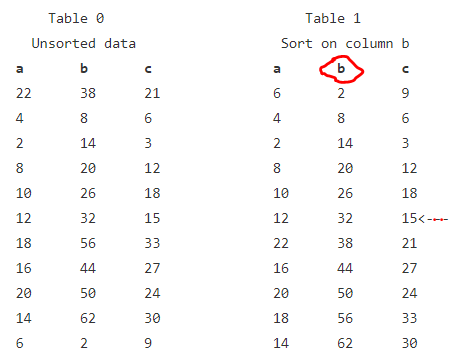In :
``````# Step-2
#-------#
# Divide the sorted table into two parts at the median value
# here the median value is 32 for column 'b',
# so (12,32,15) is the median record of the table and will be considered as root node
# now divide the table-1 into two tables at the median record
Image('kdtree_step2.PNG')``````
Out: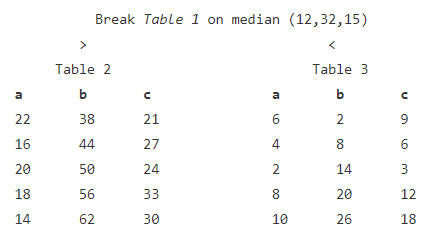In [ ]:
``````# Step-3
#-------#
# Consider these above two tables as two child nodes of the root node
# Next, from among the remaining attributes, we select that dimension that has the greatest variance
# Here out of 'a' and 'c', we see that highest variance is in column 'c'
# Again sort the tables (both) on column 'c' and break them at their respective medians
# Each of these tables will now create two more tables under them
# and will go on till you stop on achieving a certain threshold``````
In [ ]:
``````# using KD Tree
#-------------#

# Once this data structure is created, it is easy to descend down to the
# the leaf node with a bunch of records and find out the (approx) neighborhood of any point.
# The root node records can be included in any of left or right child nodes
# if there are not enough records in child nodes, the adjacent (left or right)
# child node points can also be considered
# Instead of finding the distance with all data points, KD tree is very efficient
# to find the nearest neighbors in a subset of the whole dataset
# It works best when the number of dimensions is small
# KD Tree supports only 'euclidean', 'minkowski', 'manhattan', 'chebyshev' distances``````
In :
``````#==============================#
# Ball-tree Data Structure
#==============================#

# Ball-tree is also a binary tree
# To start with, two clusters (each resembling a ball) are created
# Any point in n-dimensional space will belong to either cluster but not to both
# Next, each of the balls is again subdivided into two sub-clusters (like balls)
# We keep on dividing upto a certain path or threshold is reached and stop there``````
In [ ]:
``````# using Ball Tree
#---------------#

# An unclassified (target) point must fall within any one of the nested balls
# Then identifying k nearest neighbors in that subset of data points is a lot faster
# Ball tree data structure is very efficient in situations when number of dimensions is large
# Ball tree formation initially requires a lot of time and memory but once nested
# hyper-spheres are created and placed in memory discovery of nearest points becomes easier.
# Ball tree supports a lot more distance metrics``````
In [ ]:
``````# Both Ball Tree and KD Tree
#--------------------------#
# Both the ball tree and kd-tree implement k-neighbor and bounded neighbor
# searches, and can use either a single tree or dual tree approach, with
# either a breadth-first or depth-first tree traversal.

# Both the ball tree and kd-tree have their memory pre-allocated entirely
# by numpy: this not only leads to code that's easier to debug and
# maintain (no memory errors!), but means that either data structure can be
# serialized using Python's pickle module. This is a very important feature
# in some contexts, most notably when estimators are being sent between multiple
# machines in a parallel computing framework.

# Both the ball tree and kd-tree implement fast kernel density estimation (KDE),
# which can be used within any of the valid distance metrics. The supported kernels are
# ['gaussian', 'tophat', 'epanechnikov', 'exponential', 'linear', 'cosine']``````
In :
``````#==============================#
# Some more Insights
#==============================#
# The training phase of the kNN algorithm consists only of storing the
# feature vectors and class labels of the training samples.
# In the testing phase, a test point is classified by assigning the label
# which are most frequent among the k training samples nearest to that
# query point – hence higher computation.

# k-NN performs much better if all of the data have the same scale

# k-NN works well with a small number of input variables (p), but struggles
# when the number of inputs is very large

# Let us discuss a situation to understand kNN's behavior
# Context is that you are doing a classification and using kNN for Predictive Modeling
# If you increase the k-Size, the boundary of a given point increases
# increasing the k, you are increasing the bias
# In case of very large value of k, we may include points from other classes
# If for k=3 it predicted class-A, it might have a different outcome if k=6
# Similarly, if you decrease the size of k to 1, predictions become less stable
# If our query point is surrounded by several class Bs and just one class A
# which is a little closer than so many other class Bs, the model will predict class A

# When you are dealing with noisy data, going ahead with small k is advisable
# However, In case of too small value of k the algorithm is very sensitive to noise
# We can choose optimal value of k with the help of cross validation (always)

# kNN suffers from the curse of high-dimensionality and overfits,
# you must either do feature selection or Dimensionality reduction

# k-NN is a memory-based approach is that the classifier immediately adapts
# as we collect new training data.

# The computational complexity for classifying new samples grows linearly with
# the number of samples in the training dataset in the worst-case scenario

# k-NN does not require an explicit training step

# You can implement a 2-NN classifier by ensembling 1-NN classifiers

# Euclidean distance treats each feature as equally important

# The training time for any value of k in kNN algorithm is the same (obvious, right?)
# 1-NN ~ 2-NN ~ 3-NN

# In cases where we are taking a majority vote (e.g. picking the mode in a
# classification problem) among labels, we usually make K an odd number to have a tiebreaker.

# The algorithm gets significantly slower as the volume of data increases
Image('knn_kvalue.png')``````
Out: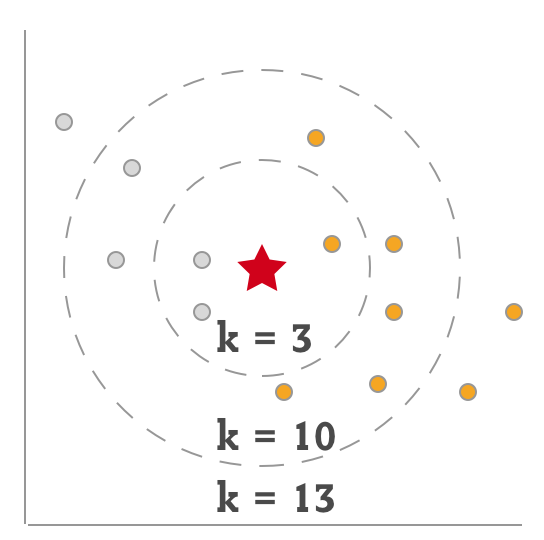In :
``````# What may happen if the data is not normalized. See the example below:
# Remember that kNN relies on the distance between the data points
Image('knn_data_normalization.png')``````
Out: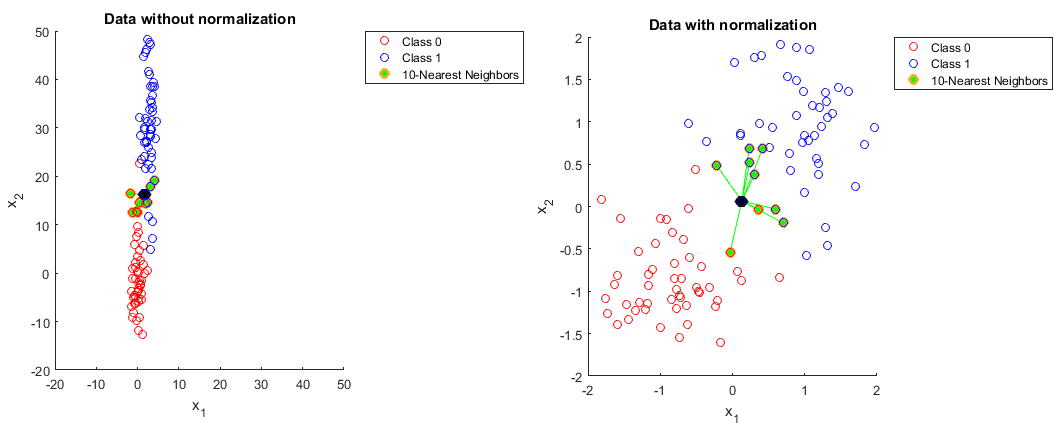In :
``````#==============================#
# kNN in Practice
#==============================#

# Since kNN gets slower with volume of data, it is not used that extensively for prediction
# There are so many good algorithms for classification and regression that scale well

# kNNs is best suitable in solving problems that have solutions that
# depend on identifying similar objects. An example of this is using the
# KNN algorithm in recommender systems, an application of KNN-search.``````

## kNN for recommender systems

In [ ]:
``````#==============================#
# Some basics
#==============================#

# Given our movies data set, what are the 5 most similar movies to a movie query?

``````
In :
``````# Assuming that we already have scaled data and divided into X_train, X_test, y_train, y_test

# We are now ready to train the kNN model for Recommendation

# Import module for KNN
from sklearn.neighbors import NearestNeighbors
# Create KNN instance
knn_rec = NearestNeighbors(n_neighbors=4, algorithm='ball_tree')
# Alternatively, one can use the KDTree or BallTree classes directly to find nearest neighbors
# kdt = KDTree(X, leaf_size=30, metric='euclidean')

# Fit (i.e. traing) the model (It can take just one argument also)
knn_rec.fit(X_train)
``````
Out:
``````NearestNeighbors(algorithm='ball_tree', leaf_size=30, metric='minkowski',
metric_params=None, n_jobs=None, n_neighbors=4, p=2, radius=1.0)``````
In :
``distances, indices = knn_rec.kneighbors(X_train)``
In :
``distances``
Out:
``````array([[0.        , 1.76734987, 1.92601215, 2.14037512],
[0.        , 1.69482904, 1.77677137, 1.80835615],
[0.        , 1.51524796, 1.78101852, 1.81458141],
...,
[0.        , 1.59471376, 1.91973144, 1.948452  ],
[0.        , 1.52628727, 1.63696573, 1.76683087],
[0.        , 1.91311964, 1.97915637, 1.99927913]])``````
In :
``indices``
Out:
``````array([[  0, 681, 729, 663],
[  1, 673, 477, 674],
[  2, 463,  90, 146],
...,
[747, 595, 494, 231],
[748, 733, 348, 715],
[749, 471, 707, 589]], dtype=int64)``````
In :
``X_train.head(2)``
Out:
In :
``````# New data point check against our k neighbors classifier.
# To search object X and identify the closest related record, we call the kneighbors() function on the new data point
print(knn_rec.kneighbors([[1.276079, 1.655702, 1.208756, 0.456246, -1.611321, 1.233389, -2.012156, 1.495924, -1.292094, 0.447591]])) ``````
```(array([[2.57262672, 2.63682582, 2.74143077, 2.83988477]]), array([[338, 666, 35, 77]], dtype=int64)) ```
In :
``indices[0:2]``
Out:
``````array([[  0, 681, 729, 663],
[  1, 673, 477, 674]], dtype=int64)``````
In [ ]:
`` ``
In [ ]:
`` ``
In [ ]:
`` ``